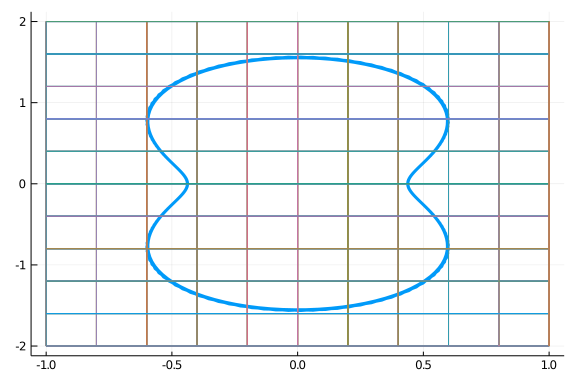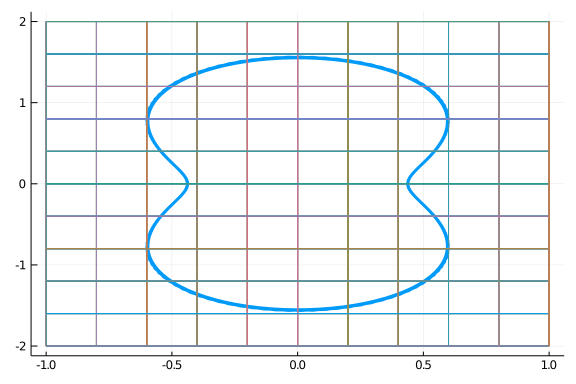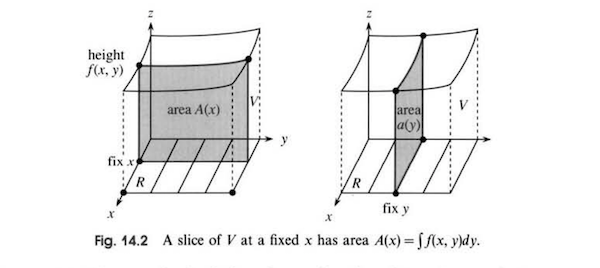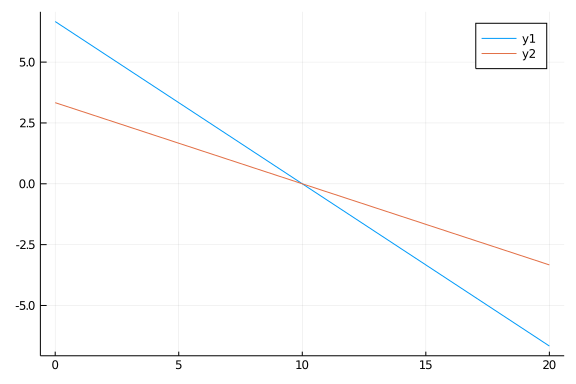# Multi-dimensional integrals

The definition of the definite integral, $\int_a^b f(x)dx$, is based on Riemann sums.

We review, using a more general form than previously. Consider a bounded function $f$ over $[a,b]$. A partition, $P$, is based on $a = x_0 < x_1 < \cdots < x_n = b$. For each subinterval $[x_{i-1}, x_{i}]$ take $m_i(f) = \inf_{u \text{ in } [x_{i-1},x_i]} f(u)$ and $M_i(f) = \sup_{u \text{ in } [x_{i-1},x_i]} f(u)$. (When $f$ is continuous, $m_i$ and $M_i$ are realized at points of $[x_{i-1},x_i]$, though that isn't assumed here. The use of "$\sup$" and "$\inf$" is a mathematically formal means to replace this in general.) Let $\Delta x_i = x_i - x_{i-1}$. Form the sums $m(f, P) = \sum_i m_i(f) \Delta x_i$ and $M(f, P) = \sum_i M_i(f) \Delta x_i$. These are the lower and upper Riemann sums for a partition. A general Riemann sum would be formed by selecting $c_i$ from $[x_{i-1}, x_i]$ and forming $S(f,P) = \sum f(c_i) \Delta x_i$. It will be the case that $m(f,P) \leq S(f,P) \leq M(f,P)$, as this is true for each sub-interval of the partition.

If, as the largest diameter ($\Delta x_i$) of the partition $P$ goes to $0$, the upper and lower sums converge to the same limit, then $f$ is called Riemann integrable over $[a,b]$. If $f$ is Riemann integrable, any Riemann sum will converge to the definite integral as the partitioning shrinks.

Continuous functions are known to be Riemann integrable, as are functions with only finitely many discontinuities, though this isn't the most general case of integrable functions, which will be stated below.

In practice, we don't typically compute integrals using a limit of a partition, though the approach may provide direction to numeric answers, as the Fundamental Theorem of Calculus relates the definite integral with an antiderivative of the integrand.

The multidimensional case will prove to be similar where a Riemann sum is used to define the value being discussed, but a theorem of Fubini will allow the computation of integrals using the Fundamental Theorem of Calculus.

Before beginning, we will use several features and packages loaded by

using CalculusWithJulia
using Plots


## Integration theoryHow to estimate the volume contained within the Chrysler Building? One way might be to break the building up into tall vertical blocks based on its skyline; compute the volume of each block using the formula of volume as area of the base times the height; and, finally, adding up the computed volumes This is the basic idea of finding volumes under surfaces using Riemann integration.Computing the volume of a nano-block construction of the Chrysler building is easier than trying to find an actual tree at the Chrysler building, as we can easily compute the volume of columns of equal-sized blocks. Riemann sums are similar.

The definition of the multi-dimensional integral is more involved then the one-dimensional case due to the possibly increased complexity of the region. This will require additional steps. The basic approach is as follows.

First, let $R = [a_1, b_1] \times [a_2, b_2] \times \cdots \times [a_n, b_n]$ be a closed rectangular region. If $n=2$, this is a rectangle, and if $n=3$, a box. We begin by defining integration over closed rectangular regions. For each side, a partition $P_i$ is chosen based on $a_i = x_{i0} < x_{i1} < \cdots < x_{ik} = b_i$. Then a sub-rectangular region would be of the form $R' = P_{1j_1} \times P_{2j_2} \times \cdots \times P_{nj_n}$, where $P_{ij_i}$ is one of the partitioning sub intervals of $[a_i, b_i]$. Set $\Delta R' = \Delta P_{1j_1} \cdot \Delta P_{2j_2} \cdot\cdots\cdot\Delta P_{nj_n}$ to be the $n$-dimensional volume of the sub-rectangular region.

For each sub-rectangular region, we can define $m(f,R')$ to be $\inf_{u \text{ in } R'} f(u)$ and $M(f, R') = \sup_{u \text{ in } R'} f(u)$. If we enumerate all the sub-rectangular regions, we can define $m(f, P) = \sum_i m(f, R_i) \Delta R_i$ and $M(f,P) = \sum_i M(f, R_i)\Delta R_i$, as in the one-dimensional case. These are upper and lower sums, and, as before, would bound the Riemann sum formed by choosing any $c_i$ in $R_i$ and computing $S(f,P) = \sum_i f(c_i) \Delta R_i$.

As with the one-dimensional case, $f$ is Riemann integrable over $R$ if the limits of $m(f,P)$ and $M(f,P)$ exist and are identical as the diameter of the partition (defined as the largest diameter of each side) goes to $0$. If the limits are equal, then so is the limit of any Riemann sum.

When $f$ is Riemann integrable over a rectangular region $R$, we denote the limit by any of:

$~ \iint_R f(x) dV, \quad \iint_R fdV, \quad \iint_R f(x_1, \dots, x_n) dx_1 \cdot\cdots\cdot dx_n, \quad\iint_R f(\vec{x}) d\vec{x}. ~$

A key fact, requiring proof, is:

Any continuous function, $f$, is Riemann integrable over a closed, bounded rectangular region.

As with one-dimensional integrals, from the Riemann sum definition, several familiar properties for integrals follow. Let $V(R)$ be the volume of $R$ found by multiplying the side-lengths together.

• A constant is Riemann integrable and: $\iint_R c dV = c V(R)$.

Linearity

• For integrable $f$ and $g$ and constants $a$ and $b$:

$~ \iint_R (af(x) + bg(x))dV = a\iint_R f(x)dV + b\iint_R g(x) dV. ~$

• If $R$ and $R'$ are disjoint rectangular regions (possibly sharing a boundary), then the integral over the union is defined by linearity:

$~ \iint_{R \cup R'} f(x) dV = \iint_R f(x)dV + \iint_{R'} f(x) dV. ~$

Monotonicity

• As $f$ is bounded, let $m \leq f(x) \leq M$ for all $x$ in $R$. Then

$~ m V(R) \leq \iint_R f(x) dV \leq MV(R). ~$

• If $f$ and $g$ are integrable and $f(x) \leq g(x)$, then the integrals have the same property, namely $\iint_R f dV \leq \iint_R gdV$.

• If $S \subset R$, both closed rectangles, then if $f$ is integrable over $R$ it will be also over $S$ and, when $f\geq 0$, $\iint_S f dV \leq \iint_R fdV$.

• If $f$ is bounded and integrable, then $|\iint_R fdV| \leq \iint_R |f| dV$.

### HCubature

To numerically compute multidimensional integrals over rectangular regions in Julia is efficiently done with the HCubature package. The hcubature function is defined for $n$-dimensional integrals, so the integrand is specified through a function which takes a vector as an input. The region to integrate over is of rectangular form. It is specified by a tuple of left endpoints and a tuple of right endpoints. The order is in terms of the order of the vector.

To elaborate, if we think of $f(\vec{x}) = f(x_1, x_2, \dots, x_n)$ and we are integrating over $[a_1, b_1] \times \cdots \times [a_n, b_n]$, then the region would be specified through two tuples: (a1, a2, ..., an) and (b1, b2, ..., bn).

To illustrate, to integrate the function $f(x,y) = x^2 + 5y^2$ over the region $[0,1] \times [0,2]$ using HCubature's hcubature function, we would proceed as follows:

using HCubature  # loaded by CalculusWithJulia

f(x,y) = x^2 + 5y^2
f(v) = f(v...)  # f accepts a vector
a0, b0 = 0, 1
a1, b1 = 0, 2
hcubature(f, (a0, a1), (b0, b1))

(14.0, 1.7763568394002505e-15)


The computed value and a worst case estimate for the error is returned, in a manner similar to the quadgk function (from the QuadGK package) used previously for one-dimensional numeric integrals.

The order above is x then y, which is clear from the first definition of f and as belabored in the tuples passed to hcubature. A more convenient use is to just put the constants into the function call, as in hcubature(f, (0,0), (1,2)).

##### Example

Let's verify the numeric approach works for figures where an answer is known from the geometry of the problem.

• A constant function $c=f(x,y)$. In this case, the volume is simply a box, so the volume will come from multiplying the three dimensions. Here is an example:

f(x,y) = 3
f(v) = f(v...)
a0, b0 = 0, 4
a1, b1 = 0, 5  # R is area 20, so V = 60 = 3 ⋅ 20
hcubature(f, (a0, a1), (b0, b1))

(60.0, 7.105427357601002e-15)

• A wedge. Let $f(x,y) = x$ and $R= [0,1] \times [0,1]$. The the volume is a wedge, and should be half the value of the unit cube, or simply $1/2$:

f(x,y) = x
f(v) = f(v...)
a0, b0 = 0, 1
a1, b1 = 0, 1
hcubature(f, (a0, a1), (b0, b1))

(0.5, 0.0)

• The volume of a right square pyramid is $V=(1/3)a^2 h$, or a third of an enclosing box. We computed this area previously using the method of slices. Here we do it thinking of the pyramid as the volume formed by the surface over the region $[-a,a] \times [-a,a]$ generated by $f(x,y) = h \cdot (l(x,y) - d(x,y))/l(x,y)$ where $d(x,y)$ is the distance to the origin, or $\sqrt{x^2 + y^2}$ and $l(x,y)$ is the length of the line segment from the origin to the boundary of $R$ that goes through $(x,y)$.

Identifying a formula for this is a bit tricky. Here we use a brute force approach; later we will simplify this. Using polar coordinates, we know $r\cos(\theta) = a$ describes the line $x=a$ and $r\sin(\theta)=a$ describes the line $y=a$. Using the square, we have to alternate between these depending on where $\theta$ is (e.g., between $-\pi/4$ and $\pi/4$ it would be $r\cos(\theta)=a$ or $a/\cos(\theta)$ is $l(x,y)$. We write a function for this:

d(x, y)  = sqrt(x^2 + y^2)
function l(x, y, a)
theta = atan(y,x)
atheta = abs(theta)
if (pi/4 <= atheta < 3pi/4) # this is the y=a or y=-a case
(a/2)/sin(atheta)
else
(a/2)/abs(cos(atheta))
end
end

l (generic function with 1 method)


And then

f(x,y,a,h) = h * (l(x,y,a) - d(x,y))/l(x,y,a)
a, height = 2, 3
f(v) = f(v,v, a, height)  # fix a and h

f (generic function with 3 methods)


We can visualize the volume to be computed, as follows:

f(x,y) = f(x,y, a, height)
xs = ys = range(-1, 1, length=20)
surface(xs, ys, f)Trying this, we have:

hcubature(f, (-a/2, -a/2), (a/2, a/2))

(4.000000009419285, 5.9590510310780164e-8)


The answer agrees with that known from the formula, $4 = (1/3)a^2 h$, but the answer takes a long time to be produce. The hcubature function is slow with functions defined in terms of conditions. For this problem, volumes by slicing is more direct. But also symmetry can be used, were we able to compute the volume above the triangular region formed by the $x$-axis, the line $x=a/2$ and the line $y=x$, which would be $1/8$th the total volume. (As then $l(x,y,a) = (a/2)/\sin(\tan^{-1}(y,x))$.).

• The volume of a sphere is $4/3 \pi r^3$. We could verify this by integrating $z = f(x,y) = \sqrt{r^2 - (x^2 + y^2)}$ over $R = \{(x,y): x^2 + y^2 \leq r^2\}$. However, this is not a rectangular region, so we couldn't directly proceed.

We might try integrating a function with a condition:

function f(x,y, r)
if x^2 + y^2 < r
sqrt(z - x^2 + y^2)
else
0.0
end
end

f (generic function with 4 methods)


But hcubature is very slow to integrate such functions. We will see our instincts are good – this is the approach taken to discuss integrals over general regions – but this is not practical here. There are two alternative approaches to be discussed: approach the integral iteratively or transform the circular region into a rectangular region and integrate. Before doing so, we discuss how the integral is developed for more general regions.

## Integrals over more general regions

To proceed further, it is necessary to discuss certain types of sets that will be used to describe the boundaries of regions that can be integrated over, though we don't dig into the details.

Let the measure of a rectangular region be its volume and for any subset of $S \subset R^n$, define the outer measure of $S$ by $m^*(S) = \inf\sum_{j=1}^\infty V(R_j)$ where the infimum is taken over all closed, countable, rectangles with $S \subset \cup_{j=1}^\infty R_j$.

In two dimensions, if $S$ is viewed on a grid, then this would be area of the smallest collection of cells that contain any part of $S$. This is the smallest this value takes as the grid becomes infinite.

For the following graph, there are $100$ cells each of area $8/100$. Their are 58 cells covering the curve and its interior. So the outer measure is less than $58\cdot 8/100$, as this is just one possible covering.A set has measure $0$ if the outer measure is $0$. An alternate definition, among other characterizations, is a set has measure $0$ if for any $\epsilon > 0$ there exists rectangular regions $R_1, R_2, \dots, R_n$ (for some $n$) with $\sum V(R_i) < \epsilon$. Measure zero sets have many properties not discussed here.

For now, let's see that graph of $y=f(x)$ over $[a,b]$, as a two dimensional set, has measure zero when $f(x)$ has a bounded derivative ($|f'|$ bounded by $M$). Fix some $\epsilon>0$. Take $n$ with $2M(b-a)^2/n < \epsilon$, then divide $[a,b]$ into $n$ equal length intervals (of length $\delta = (b-a)/n)$. For each interval, we consider the box $[a_i, b_i] \times [f(a_i)-\delta M, f(a_i) + \delta M]$. By the mean value theorem, we have $|f(x) - f(a_i)| \leq |b_i-a_i|M$ so $f(a_i) - \delta M \leq f(x) \leq f(a_i) + \delta M$, so the curve will stay in the boxes. These boxes have total area $n \cdot \delta \cdot 2\delta M = 2M(b-a)^2/n$, an area less than $\epsilon$.

The above can be extended to any graph of a continuous function over $[a,b]$.

For a function $f$ the set of discontinuities in $R$ is all points where $f$ is not continuous. A formal definition is often given in terms of oscillation. Let $o(f, \vec{x}, \delta) = \sup_{\{\vec{y} : \| \vec{y}-\vec{x}\| < \delta\}}f(\vec{y}) - \inf_{\{\vec{y}: \|\vec{y}-\vec{x}\|<\delta\}}f(\vec{y})$. A function is discontinuous at $\vec{x}$ if the limit as $\delta \rightarrow 0+$ (which must exist) is not $0$.

With this, we can state the Riemann-Lebesgue theorem on integrable functions:

Let $R$ be a closed, rectangular region, and $f:R^n \rightarrow R$ a bounded function. Then $f$ is Riemann integrable over $R$ if and only if the set of discontinuities is a set of measure $0$.

It was said at the outset we would generalize the regions we can integrate over, but this theorem generalizes the functions. We can tie the two together as follows. Define the integral over any bounded set $S$ with boundary of measure $0$. Bounded means $S$ is contained in some bounded rectangle $R$. Let $f$ be defined on $S$ and extend it to be $0$ on points in $R$ that are not in $S$. If this extended function is integrable over $R$, then we can define the integral over $S$ in terms of that. This is why the boundary of $S$ must have measure zero, as in general it is among the set of discontinuities of the extend function $f$. Such regions are also called Jordan regions.

## Fubini's theorem

Consider again this figureLet $C_i$ enumerate all the cells shown, assume $f$ is extended to be $0$ outside the region, and let $c_i$ be a point in the cell. Then the Riemann sum $\sum_i f(c_i) V(C_i)$ can be visualized three identical ways:

• as a linear sum over the indices $i$, as written, leading to $\iint_R f(x) dV$.

• by indexing the cells by row ($i$) and column ($j$) and summing as $\sum_i (\sum_j f(x_{ij}, y_{ij}) \Delta y_j) \Delta x_i$.

• by indexing the cells by row ($i$) and column ($j$) and summing as $\sum_j (\sum_i f(x_{ij}, y_{ij}) \Delta x_i) \Delta y_j$.

The last two suggest that their limit will be iterated integrals of the form $\int_{-1}^1 (\int_{-2}^2 f(x,y) dy) dx$ and $\int_{-2}^2 (\int_{-1}^1 f(x,y) dx) dy$.

By "iterated" we mean performing two different definite integrals. For example, to compute $\int_{-1}^1 (\int_{-2}^2 f(x,y) dy) dx$ the first task would be to compute $I(x) = \int_{-2}^2 f(x,y) dy$. Like partial derivatives, this integrates in $y$ while treating $x$ as a constant. Once the interior integral is computed, then the integral $\int_{-1}^1 I(x) dx$ would be computed to find the answer.

The question then: under what conditions will the three integrals be equal?

Fubini. Let $R \times S$ be a closed rectangular region in $R^n \times R^m$. Suppose $f$ is bounded. Define $f_x(y) = f(x,y)$ and $f^y(x) = f(x,y)$ where $x$ is in $R^n$ and $y$ in $R^m$. If $f_x$ and $f^y$ are integrable then $~ \iint_{R\times S}fdV = \iint_R \left(\iint_S f_x(y) dy\right) dx = \iint_S \left(\iint_R f^y(x) dx\right) dy. ~$

Similarly, if $f^y$ is integrable for all $y$, then $\iint_{R\times S}fdV =\iint_S \iint_R f(x,y) dx dy$.

An immediate corollary is that the above holds for continuous functions when $R$ and $S$ are bounded, the case described here.

The case of continuous functions was known to Euler, Lebesgue (1904) discussed bounded functions, as in our statement, and Fubini and Tonnelli (1907 and 1909) generalized the statement to more general functions than continuous functions, thereby earning naming rights.

In Ferzola we can read a summary of Euler's thinking of 1769 when trying to understand the integral of a function $f(x,y)$ over a bounded domain $R$ enclosed by arcs in the $x$-$y$ plane. (That is, the area below $g(x)$ and above $h(x)$ over the interval $[a,b]$.) Euler wrote the answer as $\int_a^b dx (\int_{g(x)}^{h(x)} f(x,y)dy)$. Ferzola writes that Euler saw this integral yielding a volume as the integral $\int_{g(x)}^{h(x)} f(x,y)dy$ gives the area of a slice (parallel to the $y$ axis) and integrating in $x$ adds these slices to give a volume. This is the typical usage of Fubini's theorem today.Figure 14.2 of Strang illustrating the slice when either $x$ is fixed or $y$ is fixed. The inner integral computes the shared area, the outer integral adds the areas up to compute volume.

In Volumes the formula for a volume with a known cross-sectional area is given by $V = \int_a^b CA(x) dx$. The inner integral, $\int_{R_x} f(x,y) dy$ is a function depending on $x$ that yields the area of the slice (where $R_x$ is the region sliced by the line of constant $x$ value). This is consistent with Euler's view of the iterated integral.

A domain, as described above, is known as a normal domain. Using Fubini's theorem to integrate iteratively, employing the fundamental theorem of calculus at each step, is the standard approach.

For example, we return to the problem of a square pyramid, only now using symmetry, we integrate only over the triangular region between $0 \leq x \leq a/2$ and $0 \leq y \leq x$. The answer is then (the $8$ by symmetry)

$~ V = 8 \int_0^{a/2} \int_0^x h(l(x,y) - d(x,y))/l(x,y) dy dx. ~$

But, using similar triangles, we have $d/x = l/(a/2)$ so $(l-d)/l = 1 - 2x/a$. Continuing, our answer becomes

$~ V = 8 \int_0^{a/2} (\int_0^x h(1-\frac{2x}{a}) dy) dx = 8 \int_0^{a/2} (h(1-2x/a) \cdot x) dx = 8 (hx^2_2 \big\lvert_{0}^{a/2} - \frac{2}{a}\frac{x^3}{3}\big\lvert_0^{a/2})= 8 h(\frac{a^2}{8} - \frac{2}{24}a^2) = \frac{a^2h}{3}. ~$

### SymPy's integrate

The integrate function of SymPy uses various algorithms to symbolically integrate definite (and indefinite) integrals. In the section on integrals its use for one-dimensional integrals was shown. For multi-dimensional integrals the usage is similar, the syntax following, somewhat, the Fubini-like notation.

For example, to perform the integral

$~ \int_a^b \int_{h(x)}^{g(x)} f(x,y) dy dx ~$

the call would look like:

integrate(f(x,y), (y, h(x), g(x)), (x, a, b))


That is, the variable to integrate and the endpoints are passed as tuples. (Unlike hcubature which always uses two tuples to specify the bounds, integrate uses $n$ tuples to specify an $n$-dimensional integral.) The iteration happens from left to write, so in the above the y integral is done (and, as seen, may depend on the variable x) and then the x integral is performed. The above uses f(x,y), h(x) and g(x), but these may be simple symbolic expressions and not function calls using symbolic variables.

##### Example

For example, the last integral to compute the volume of a square pyramid, could be computed through

@vars x y a height
8 * integrate(height * (1 - 2x/a), (y, 0, x), (x, 0, a/2))

\begin{equation*}\frac{a^{2} height}{3}\end{equation*}
##### Example

Find the integral $\int_0^1\int_{y^2}^1 y \sin(x^2) dx dy$.

Without concerning ourselves with what or why, we just translate:

@vars x y
integrate( y * sin(x^2), (x, y^2, 1), (y, 0, 1))

\begin{equation*}- \frac{3 \sqrt{2} \sqrt{\pi} \left(\frac{3 \sqrt{2} \cos{\left(1 \right)} \Gamma\left(\frac{3}{4}\right)}{16 \sqrt{\pi} \Gamma\left(\frac{7}{4}\right)} + \frac{3 S\left(\frac{\sqrt{2}}{\sqrt{\pi}}\right) \Gamma\left(\frac{3}{4}\right)}{8 \Gamma\left(\frac{7}{4}\right)}\right) \Gamma\left(\frac{3}{4}\right)}{8 \Gamma\left(\frac{7}{4}\right)} + \frac{3 \sqrt{2} \sqrt{\pi} S\left(\frac{\sqrt{2}}{\sqrt{\pi}}\right) \Gamma\left(\frac{3}{4}\right)}{16 \Gamma\left(\frac{7}{4}\right)} + \frac{9 \Gamma^{2}\left(\frac{3}{4}\right)}{64 \Gamma^{2}\left(\frac{7}{4}\right)}\end{equation*}
##### Example

Find the volume enclosed by $y = x^2$, $y = 5$, $z = x^2$, and $z = 0$.

The limits on $z$ say this is the volume under the surface $f(x,y) = x^2$, over the region defined by $y=5$ and $y = x^2$. The region is a parabola with $y$ running from $x^2$ to $5$, while $x$ ranges from $-\sqrt{5}$ to $\sqrt{5}$.

f(x, y) = x^2
h(x) = x^2
g(x) = 5
integrate(f(x,y), (y, h(x), g(x)), (x, -sqrt(Sym(5)), sqrt(Sym(5))))

\begin{equation*}\frac{20 \sqrt{5}}{3}\end{equation*}
##### Example

Find the volume above the $x$-$y$ plane when a cylinder, $x^2 + y^2 = 2^2$ is intersected by a plane $3x + 4y + 5z = 6$.

We solve for $z = (1/5)\cdot(6 - 3x - 4y)$ and take $R$ as the disk at the origin of radius $2$:

f(x,y) = 6 - 3x - 4y
g(x) = sqrt(2^2 - x^2)
h(x) = -sqrt(2^2 - x^2)
(1//5) * integrate(f(x,y), (y, h(x), g(x)), (x, -2, 2))

\begin{equation*}\frac{24 \pi}{5}\end{equation*}
##### Example

Find the volume:

• in the first octant

• bounded by $x+y+z = 10$, $2x + 3y = 20$, and $x + 3y = 10$

The first plane can be expressed as $z = f(x,y) = 10 - x - y$ and the volume is that below the surface of $f$ over the region $R$ formed by the two lines and the $x$ and $y$ axes. Plotting that we have:

g1(x) = (20 - 2x)/3
g2(x) = (10 - x)/3
plot(g1, 0, 20)
plot!(g2, 0, 20)We see the intersection is when $x=10$, so this becomes

f(x,y) = 10 - x - y
h(x) = (10 - x)/3
g(x) = (20 - 3x)/3
integrate(f(x,y), (y, h(x), g(x)), (x, 0, 10))

\begin{equation*}\frac{500}{27}\end{equation*}
##### Example

Let $r=1$ and define three cylinders along the $x$, $y$, and $z$ axes by: $y^2+z^2 = r^2$, $x^2 + z^2 = r^2$, and $x^2 + y^2 = r^2$. What is the enclosed volume?

Using the cylinder along the $z$ axis, we have the volume sits above and below the disk $R = x^2 + y^2 \leq r^2$. By symmetry, we can double the volume that sits above the disk to answer the question.

Using symmetry, we can tell that the the wedge between $x=0$, $y=x$, and $x^2 + y^2 \leq 1$ (corresponding to a polar angle in $[0,\pi/4]$ in $R$ contains $1/8$ the volume of the top, so $1/16$ of the total.